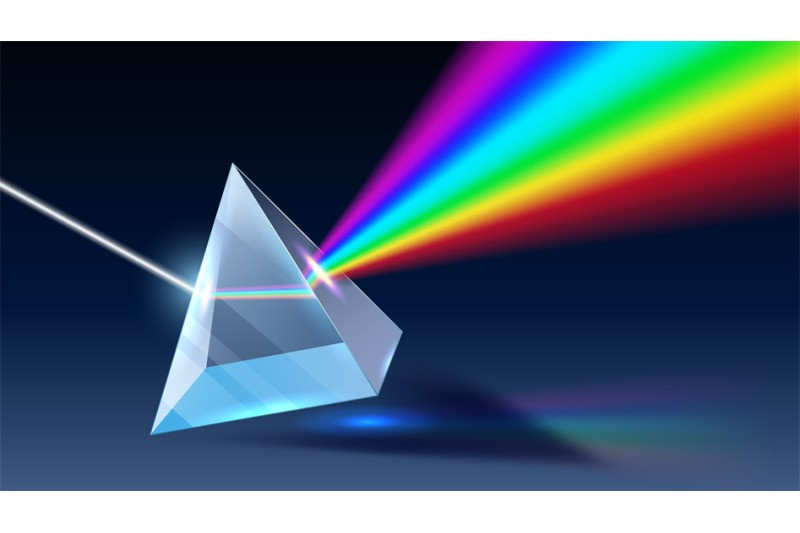## Refraction through a Prism

ABSTRACT

Prism is a homogenous , transparent medium bounded by two plane surfaces, inclined to each other at an angle.

It has two refractive surfaces,  angle of the prism , refractive edge and the principle section of the prism.

A prism transmits light but slows it down. When light passes from air to the glass of the prism, the change in speed causes the light to change direction and bend. Due to the differences in the refraction index between the air and the glass, light bends once entering the prism. The image thus formed on a screen is called the spectrum. The principle colours are given by the word VIBGYOR.

OBJECTIVE :  Refraction through a Prism.

REASONS

Refraction is the bending of light when it goes from one medium to another medium, when a ray of light passes through a glass prism, refraction of light occurs both, when it enters the prism as well as when it leaves the prism. Since the refracting surfaces are not parallel, therefore, the emergent ray and incident ray are not parallel to one another. In this case the ray of light is deviated on passing through the prism.

INTRODUCTION

In 1665, Isaac Newton discovered that white light consists of seven colours. He found that if a beam of white light is passed through glass prism then it will split in to seven colours. These colours are red, orange, yellow, green, blue, indigo and violet (VIBGYOR).

Spectrum of white light: The band of seven colours formed when a beam of white light is passes through a glass prism is called spectrum of white light.

Dispersion of light: The splitting of white light into seven colours on passing through a transparent medium is called dispersion of light.

The inclined angle of the prism is important for the refraction of light in the optical medium:

• Refracting angle or apical angle of a prism is the angle between two surfaces. The greater the angle formed by two surfaces at the apex, the strongest the prismatic effect.
• Axis of the prism refers to the line bisecting the apical angle.
• Base of the prism refers to the surface opposite to apical angle. When prescribing a prism, the orientation is indicated by the position of the base
•  e.g ‘base in’ or base out’ or base up’or ‘base down’

When a light ray strikes the surface of a dispersing prism, it is refracted upon entering according to Snell’s law and then passes through the glass until the second interface is reached. Once again, the light ray is refracted and are deviated towards the base of the prism. The prism produces displacement of the object seen through its towards apex.

The lens is formed by two prisms, like

• Concave lens ( minus lens ) are formed by two prisms where the apex meet at the centre point of the lens and base is in other side of each other.
• Convex lens ( plus lens ) are formed by two prisms where the base meet at the centre point of the lens and apex is in other side of each other.

Refraction through the prism depends on:

1. Angle of deviation(D): refers to the net change in direction of the light passing through the prism. It is determined by three factors:
1. Refractive Index of the prism material ,
2. Refracting angle of the prism,
3. Angle of incidence of the ray.
1. Angle of minimum deviation: The minimum value of the angle of deviation suffered by the ray of light on passing through a prism is called the angle of minimum deviation and is denoted by  δm.

Minimum deviation is possible when the angle of incidence is equal to the angle of emergence.(I = e)

3. The image formed by a prism is erect, virtual and displaced towards the apex of the prism.Deviation is also reduced to a minimum, when light passes through the prism symmetrically.

4. Power of a prism is measured in prism dioptres. One prism dioptre produces displacement of an object by 1cm, when kept at  a distance of 1m. One prism dioptre of displacement is central.

### Moutusi Saha

Healthcare and Humanity goes hand in hand.